### Home > CC4 > Chapter 11 > Lesson 11.2.6 > Problem11-131

11-131.
1. Solve the equations and inequality below. Check your solution(s), if possible. Explain your steps for part (c). Homework Help ✎

2.  a. 300x − 1500 = 2400 b.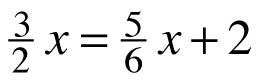c.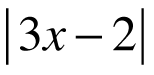> 4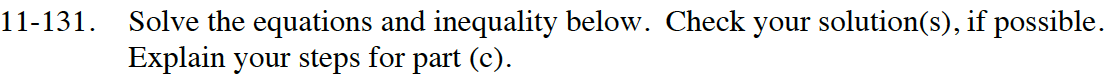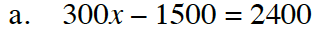What is the largest number you can divide all three terms by?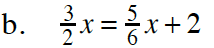What can you multiply all three terms by to get rid of the fractions?

x = 3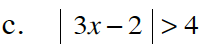Set up two equations and solve for x.

Test numbers to determine the solution regions.

$\textit{x}<-\frac{2}{3}$

or x > 2